This book is archived and will be removed July 6, 2022. Please use the updated version.

Particle Physics and Cosmology

# 87 Evolution of the Early Universe

### Learning Objectives

By the end of this section, you will be able to:

• Describe the evolution of the early universe in terms of the four fundamental forces
• Use the concept of gravitational lensing to explain astronomical phenomena
• Provide evidence of the Big Bang in terms of cosmic background radiation
• Distinguish between dark matter and dark energy

In the previous section, we discussed the structure and dynamics of universe. In particular, the universe appears to be expanding and even accelerating. But what was the universe like at the beginning of time? In this section, we discuss what evidence scientists have been able to gather about the early universe and its evolution to present time.

### The Early Universe

Before the short period of cosmic inflation, cosmologists believe that all matter in the universe was squeezed into a space much smaller than an atom. Cosmologists further believe that the universe was extremely dense and hot, and interactions between particles were governed by a single force. In other words, the four fundamental forces (strong nuclear, electromagnetic, weak nuclear, and gravitational) merge into one at these energies ((Figure)). How and why this “unity” breaks down at lower energies is an important unsolved problem in physics.

The separation of the four fundamental forces in the early universe.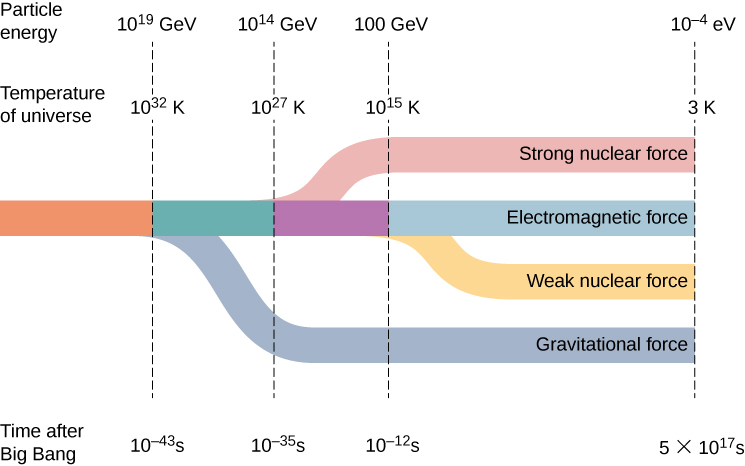Scientific models of the early universe are highly speculative. (Figure) shows a sketch of one possible timeline of events.

An approximate timeline for the evolution of the universe from the Big Bang to the present.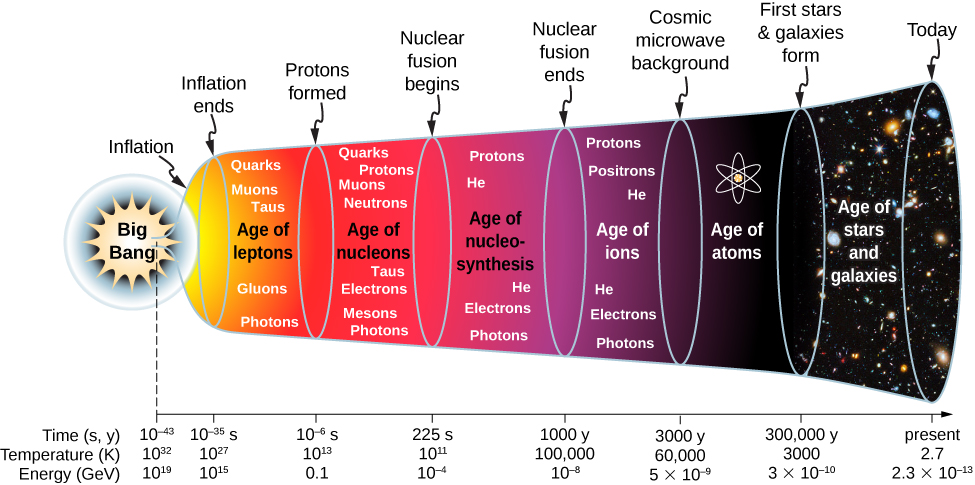1. Big Bang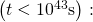The current laws of physics break down. At the end of the initial Big Bang event, the temperature of the universe is approximately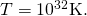2. Inflationary phase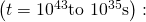The universe expands exponentially, and gravity separates from the other forces. The universe cools to approximately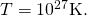3. Age of leptons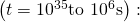As the universe continues to expand, the strong nuclear force separates from the electromagnetic and weak nuclear forces (or electroweak force). Soon after, the weak nuclear force separates from the electromagnetic force. The universe is a hot soup of quarks, leptons, photons, and other particles.
4. Age of nucleons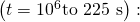The universe consists of leptons and hadrons (such as protons, neutrons, and mesons) in thermal equilibrium. Pair production and pair annihilation occurs with equal ease, so photons remain in thermal equilibrium: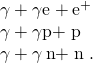The number of protons is approximately equal to the number of neutrons through interactions with neutrinos: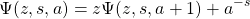The temperature of the universe settles to approximately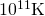—much too cool for the continued production of nucleon-antinucleon pairs. The numbers of protons and neutrons begin to dominate over their anti-particles, so proton-antiproton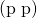and neutron-antineutron (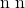) annihilations decline. Deuterons (proton-neutron pairs) begin to form.

5. Age of nucleosynthesis (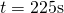to 1000 years): As the universe continues to expand, deuterons react with protons and neutrons to form larger nuclei; these larger nuclei react with protons and neutrons to form still larger nuclei. At the end of this period, about 1/4 of the mass of the universe is helium. (This explains the current amount of helium in the universe.) Photons lack the energy to continue electron-positron production, so electrons and positrons annihilate each other to photons only.
6. Age of ions (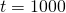to 3000 years): The universe is hot enough to ionize any atoms formed. The universe consists of electrons, positrons, protons, light nuclei, and photons.
7. Age of atoms (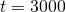to 300,000 years): The universe cools below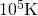and atoms form. Photons do not interact strongly with neutral atoms, so they “decouple” (separate) from atoms. These photons constitute the cosmic microwave background radiation to be discussed later.
8. Age of stars and galaxies (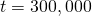years to present): The atoms and particles are pulled together by gravity and form large lumps. The atoms and particles in stars undergo nuclear fusion reaction.

To describe the conditions of the early universe quantitatively, recall the relationship between the average thermal energy of particle (E) in a system of interacting particles and equilibrium temperature (T) of that system: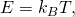where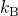is Boltzmann’s constant. In the hot conditions of the early universe, particle energies were unimaginably large.

What Was the Average Thermal Energy of a Particle just after the Big Bang?

Strategy The average thermal energy of a particle in a system of interacting particles depends on the equilibrium temperature of that system (Figure). We are given this approximate temperature in the above timeline.

Solution Cosmologists think the temperature of the universe just after the Big Bang was approximatelyTherefore, the average thermal energy of a particle would have been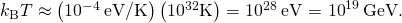Significance This energy is many orders of magnitude larger than particle energies produced by human-made particle accelerators. Currently, these accelerators operate at energies less than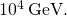Check Your Understanding Compare the abundance of helium by mass 10,000 years after the Big Bang and now.

Nucleons form at energies approximately equal to the rest mass of a proton, or 1000 MeV. The temperature corresponding to this energy is therefore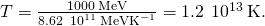Temperatures of this value or higher existed within the first second of the early universe. A similar analysis can be done for atoms. Atoms form at an energy equal to the ionization energy of ground-state hydrogen (13 eV). The effective temperature for atom formation is therefore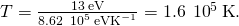This occurs well after the four fundamental forces have separated, including forces necessary to bind the protons and neutrons in the nucleus (strong nuclear force), and bind electrons to the nucleus (electromagnetic force).

### Nucleosynthesis of Light Elements

The relative abundances of the light elements hydrogen, helium, lithium, and beryllium in the universe provide key evidence for the Big Bang. The data suggest that much of the helium in the universe is primordial. For instance, it turns out that that 25% of the matter in the universe is helium, which is too high an abundance and cannot be explained based on the production of helium in stars.

How much of the elements in the universe were created in the Big Bang? If you run the clock backward, the universe becomes more and more compressed, and hotter and hotter. Eventually, temperatures are reached that permit nucleosynthesis, the period of formation of nuclei, similar to what occurs at the core of the Sun. Big Bang nucleosynthesis is believed to have occurred within a few hundred seconds of the Big Bang.

How did Big Bang nucleosynthesis occur? At first, protons and neutrons combined to form deuterons,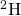. The deuteron captured a neutron to form triton,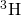—the nucleus of the radioactive hydrogen called tritium. Deuterons also captured protons to make helium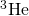. Whencaptures a proton orcaptures a neutron, helium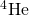results. At this stage in the Big Bang, the ratio of protons to neutrons was about 7:1. Thus, the process of conversion toused up almost all neutrons. The process lasted about 3 minutes and almost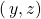of all the matter turned into, along with small percentages of,, and. Tiny amounts of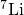and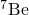were also formed. The expansion during this time cooled the universe enough that the nuclear reactions stopped. The abundances of the light nuclei,, andcreated after the Big Bang are very dependent on the matter density.

The predicted abundances of the elements in the universe provide a stringent test of the Big Bang and the Big Bang nucleosynthesis. Recent experimental estimates of the matter density from the Wilkinson Microwave Anisotropy Probe (WMAP) agree with model predictions. This agreement provides convincing evidence of the Big Bang model.

According to cosmological models, the Big Bang event should have left behind thermal radiation called the cosmic microwave background radiation (CMBR). The intensity of this radiation should follow the blackbody radiation curve (Photons and Matter Waves). Wien’s law states that the wavelength of the radiation at peak intensity is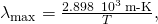where T is temperature in kelvins. Scientists expected the expansion of the universe to “stretch the light,” and the temperature to be very low, so cosmic background radiation should be long-wavelength and low energy.

In the 1960s, Arno Penzias and Robert Wilson of Bell Laboratories noticed that no matter what they did, they could not get rid of a faint background noise in their satellite communication system. The noise was due to radiation with wavelengths in the centimeter range (the microwave region). Later, this noise was associated with the cosmic background radiation. An intensity map of the cosmic background radiation appears in (Figure). The thermal spectrum is modeled well by a blackbody curve that corresponds to a temperature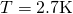((Figure)).

This map of the sky uses color to show fluctuations, or wrinkles, in the cosmic microwave background observed with the WMAP spacecraft. The Milky Way has been removed for clarity. Red represents higher temperature and higher density, whereas blue indicates lower temperature and density. This map does not contradict the earlier claim of smoothness because the largest fluctuations are only one part in one million. (credit: NASA/WMAP Science Team)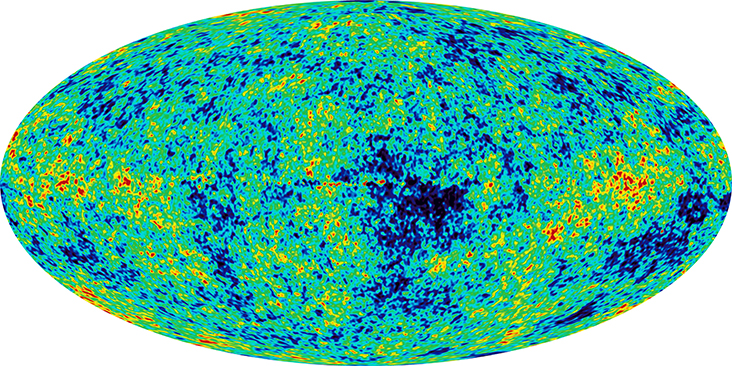Intensity distribution of cosmic microwave background radiation. The model predictions (the line) agree extremely well with the experimental results (the dots). Frequency and brightness values are shown on a log axis. (credit: modification of work by George Smoot/NASA COBE Project)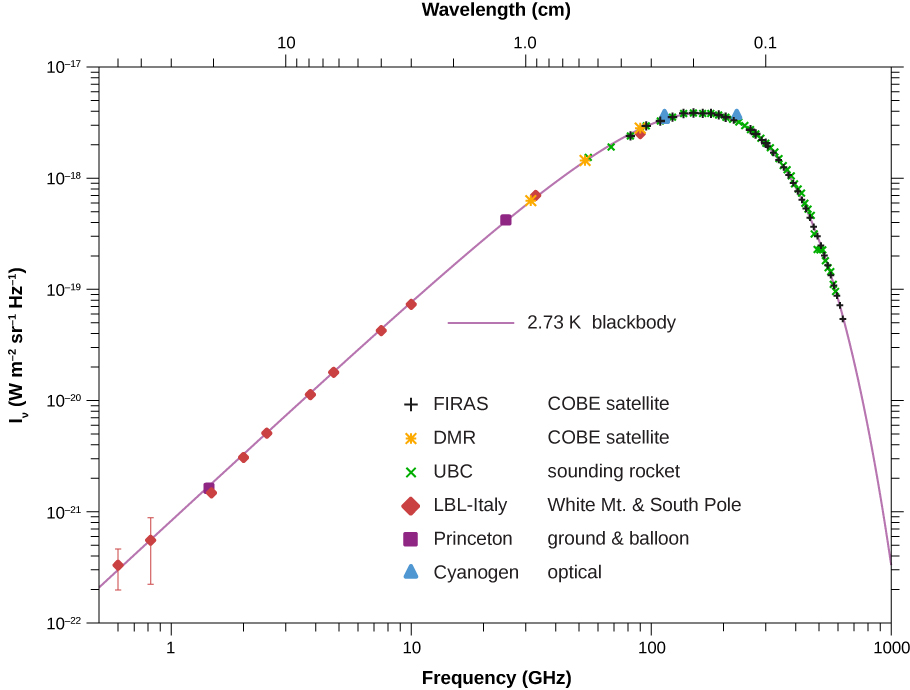The formation of atoms in the early universe makes these atoms less likely to interact with light. Therefore, photons that belong to the cosmic background radiation must have separated from matter at a temperature T associated with 1 eV (the approximate ionization energy of an atom) . The temperature of the universe at this point was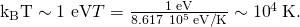According to cosmological models, the time when photons last scattered oﬀ charged particles was approximately 380,000 years after the Big Bang. Before that time, matter in the universe was in the plasma form and the photons were “thermalized.”

### Antimatter and Matter

We know from direct observation that antimatter is rare. Earth and the solar system are nearly pure matter, and most of the universe also seems dominated by matter. This is proven by the lack of annihilation radiation coming to us from space, particularly the relative absence of 0.511-MeV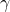rays created by the mutual annihilation of electrons and positrons. (Antimatter in nature is created in particle collisions and in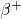decays, but only in small amounts that quickly annihilate, leaving almost pure matter surviving.)

Despite the observed dominance of matter over antimatter in the universe, the Standard Model of particle interactions and experimental measurement suggests only small differences in the ways that matter and antimatter interact. For example, neutral kaon decays produce only slightly more matter than antimatter. Yet, if through such decay, slightly more matter than antimatter was produced in the early universe, the rest could annihilate pair by pair, leaving mostly ordinary matter to form the stars and galaxies. In this way, the vast number of stars we observe may be only a tiny remnant of the original matter created in the Big Bang.

### Dark Matter and Dark Energy

In the last two decades, new and more powerful techniques have revealed that the universe is filled with dark matter. This type of matter is interesting and important because, currently, scientists do not know what it is! However, we can infer its existence by the deflection of distant starlight. For example, if light from a distant galaxy is bent by the gravitational field of a clump of dark matter between us and the galaxy, it is possible that two images of the same galaxy can be produced ((Figure)). The bending of light by the gravitational field of matter is called gravitational lensing. In some cases, the starlight travels to an observer by multiple paths around the galaxy, producing a ring ((Figure)).

Based on current research, scientist know only that dark matter is cold, slow moving, and interacts weakly with ordinary matter. Dark matter candidates include neutralinos (partners of Z bosons, photons, and Higgs bosons in “supersymmetry theory”) and particles that circulate in tiny rings set up by extra spatial dimensions.

Light from a distant star is bent around a galaxy. Under the right conditions, two duplicate images of the same star can be seen.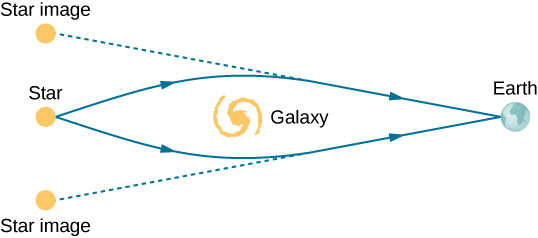Light from a distant star is bent around a galaxy. Under the right conditions, we can see a ring of light instead of a single star. (credit: modification of work by ESA/Hubble & NASA)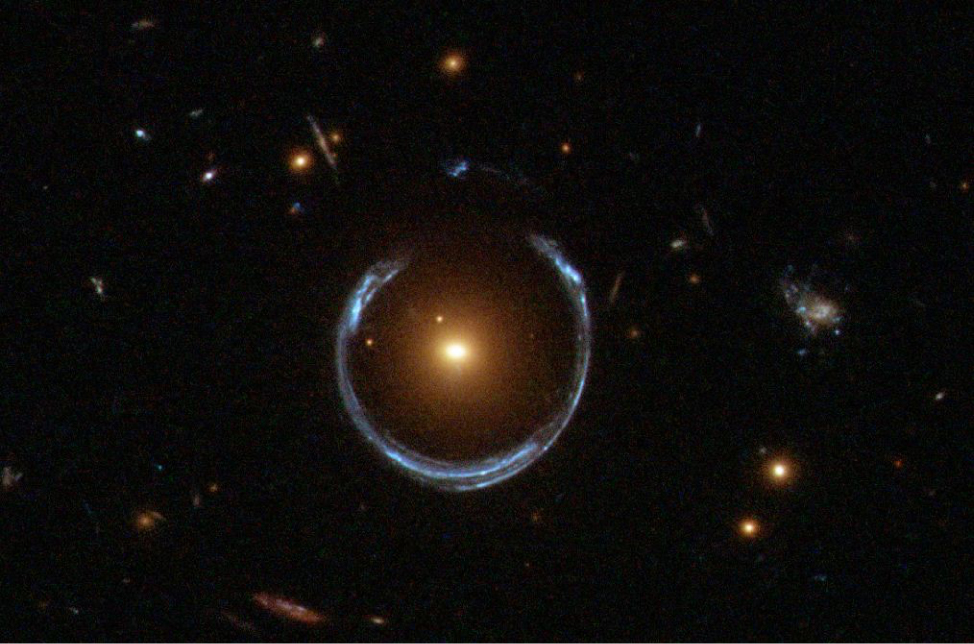Increasingly precise astronomical measurements of the expanding universe also reveal the presence of a new form of energy called dark energy. This energy is thought to explain larger-than-expected values for the observed galactic redshifts for distant galaxies. These redshifts suggest that the universe is not only expanding, but expanding at an increasing rate. Virtually nothing is known about the nature and properties of dark energy. Together, dark energy and dark matter represent two of the most interesting and unsolved puzzles of modern physics. Scientists attribute

*** QuickLaTeX cannot compile formula:
68.3\text{%}

*** Error message:
File ended while scanning use of \text@.
Emergency stop.



of the energy of the universe to dark energy,

*** QuickLaTeX cannot compile formula:
26.8\text{%}

*** Error message:
File ended while scanning use of \text@.
Emergency stop.



to dark matter, and just

*** QuickLaTeX cannot compile formula:
4.9\text{%}

*** Error message:
File ended while scanning use of \text@.
Emergency stop.



to the mass-energy of ordinary particles ((Figure)). Given the current great mystery over the nature of dark matter and dark energy, Isaac Newton’s humble words are as true now as they were centuries ago:

“I do not know what I may appear to the world, but to myself I seem to have been only like a boy playing on the sea-shore, and diverting myself in now and then finding a smoother pebble or a prettier shell than ordinary, whilst the great ocean of truth lay all undiscovered before me.”

Estimated distribution of matter and energy in the universe. (credit: NASA/WMAP Science Team)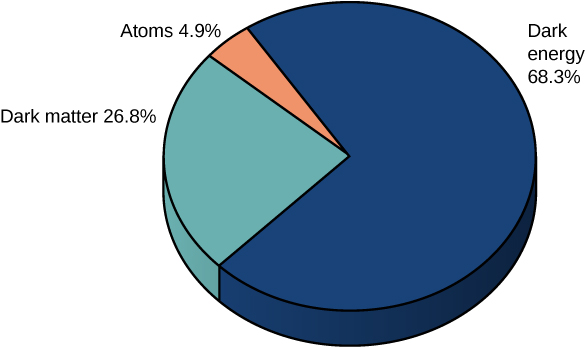### Summary

• The early universe was hot and dense.
• The universe is isotropic and expanding.
• Cosmic background radiation is evidence for the Big Bang.
• The vast portion of the mass and energy of the universe is not well understood.

### Key Equations

 Momentum of a charged particle in a cyclotron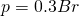Center-of-mass energy of a colliding beam machine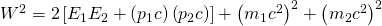Approximate time for exchange of a virtual particle between two other particles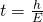Hubble’s law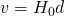Cosmological space-time metric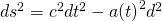### Conceptual Questions

What is meant by a “cosmological model of the early universe?” Briefly describe this model in terms of the four fundamental forces.

Describe two pieces of evidence that support the Big Bang model.

The observed expansion of the universe and the cosmic background radiation spectrum.

In what sense are we, as Newton once said, “a boy playing on the sea-shore”? Express your answer in terms of the concepts of dark matter and dark energy.

If some unknown cause of redshift—such as light becoming “tired” from traveling long distances through empty space—is discovered, what effect would that have on cosmology?

If light slow down, it takes long to reach Earth than expected. We conclude that the object is much closer than it really is. Thus, for every recessional velocity (based on the frequency of light, which we assume is not disturbed by the slowing), the distance is smaller than the “true” value, Hubble’s constant is larger than the “true” value, and the age of the universe is smaller than the “true” value.

In the past, many scientists believed the universe to be infinite. However, if the universe is infinite, then any line of sight should eventually fall on a star’s surface and the night sky should be very bright. How is this paradox resolved in modern cosmology?

Experimental results suggest that a muon decays to an electron and photon. How is this possible?

Each of the following reactions is missing a single particle. Identify the missing particle for each reaction.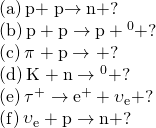a.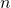; b.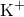; c.; d.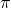; e.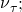f.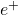Because of energy loss due to synchrotron radiation in the LHC at CERN, only 5.00 MeV is added to the energy of each proton during each revolution around the main ring. How many revolutions are needed to produce 7.00-TeV (7000 GeV) protons, if they are injected with an initial energy of 8.00 GeV?

A proton and an antiproton collide head-on, with each having a kinetic energy of 7.00 TeV (such as in the LHC at CERN). How much collision energy is available, taking into account the annihilation of the two masses? (Note that this is not significantly greater than the extremely relativistic kinetic energy.)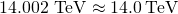When an electron and positron collide at the SLAC facility, they each have 50.0-GeV kinetic energies. What is the total collision energy available, taking into account the annihilation energy? Note that the annihilation energy is insignificant, because the electrons are highly relativistic.

The core of a star collapses during a supernova, forming a neutron star. Angular momentum of the core is conserved, so the neutron star spins rapidly. If the initial core radius is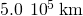and it collapses to 10.0 km, find the neutron star’s angular velocity in revolutions per second, given the core’s angular velocity was originally 1 revolution per 30.0 days.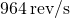Using the solution from the previous problem, find the increase in rotational kinetic energy, given the core’s mass is 1.3 times that of our Sun. Where does this increase in kinetic energy come from?

(a) What Hubble constant corresponds to an approximate age of the universe of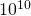y? To get an approximate value, assume the expansion rate is constant and calculate the speed at which two galaxies must move apart to be separated by 1 Mly (present average galactic separation) in a time ofy. (b) Similarly, what Hubble constant corresponds to a universe approximately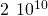years old?

a.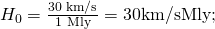b.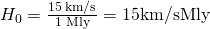### Challenge Problems

Electrons and positrons are collided in a circular accelerator. Derive the expression for the center-of-mass energy of the particle.

The intensity of cosmic ray radiation decreases rapidly with increasing energy, but there are occasionally extremely energetic cosmic rays that create a shower of radiation from all the particles they create by striking a nucleus in the atmosphere. Suppose a cosmic ray particle having an energy of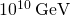converts its energy into particles with masses averaging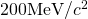.

(a) How many particles are created? (b) If the particles rain down on a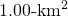area, how many particles are there per square meter?

a.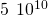; b. divide the number of particles by the area they hit: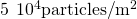(a) Calculate the relativistic quantity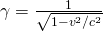for 1.00-TeV protons produced at Fermilab. (b) If such a proton created a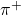having the same speed, how long would its life be in the laboratory? (c) How far could it travel in this time?

Plans for an accelerator that produces a secondary beam of K mesons to scatter from nuclei, for the purpose of studying the strong force, call for them to have a kinetic energy of 500 MeV. (a) What would the relativistic quantitybe for these particles? (b) How long would their average lifetime be in the laboratory? (c) How far could they travel in this time?

a. 2.01; b.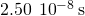; c. 6.50 m

In supernovae, neutrinos are produced in huge amounts. They were detected from the 1987A supernova in the Magellanic Cloud, which is about 120,000 light-years away from Earth (relatively close to our Milky Way Galaxy). If neutrinos have a mass, they cannot travel at the speed of light, but if their mass is small, their velocity would be almost that of light. (a) Suppose a neutrino with a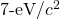mass has a kinetic energy of 700 keV. Find the relativistic quantityfor it. (b) If the neutrino leaves the 1987A supernova at the same time as a photon and both travel to Earth, how much sooner does the photon arrive? This is not a large time difference, given that it is impossible to know which neutrino left with which photon and the poor efficiency of the neutrino detectors. Thus, the fact that neutrinos were observed within hours of the brightening of the supernova only places an upper limit on the neutrino’s mass. (Hint: You may need to use a series expansion to find v for the neutrino, since itsis so large.)

Assuming a circular orbit for the Sun about the center of the Milky Way Galaxy, calculate its orbital speed using the following information: The mass of the galaxy is equivalent to a single mass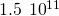times that of the Sun (or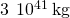), located 30,000 ly away.

*** QuickLaTeX cannot compile formula:
\begin{array}{}\\ \\ \hfill \frac{m{v}^{2}}{r}& =\hfill & \frac{GMm}{{r}^{2}}⇒\hfill \\ \\ \\ \hfill v& =\hfill & {\left(\frac{GM}{r}\right)}^{1\text{/}2}=\left[\frac{\left(6.67\phantom{\rule{0.2em}{0ex}}×\phantom{\rule{0.2em}{0ex}}{10}^{-11}\phantom{\rule{0.2em}{0ex}}\text{N}·{\text{m}}^{2}\text{/}{\text{kg}}^{2}\right)\left(3\phantom{\rule{0.2em}{0ex}}×\phantom{\rule{0.2em}{0ex}}{10}^{41}\phantom{\rule{0.2em}{0ex}}\text{kg}\right)}{\left(\text{30,000 ly}\right)\left(9.46\phantom{\rule{0.2em}{0ex}}×\phantom{\rule{0.2em}{0ex}}{10}^{15}\phantom{\rule{0.2em}{0ex}}\text{m/ly}\right)}\right]=2.7\phantom{\rule{0.2em}{0ex}}×\phantom{\rule{0.2em}{0ex}}{10}^{5}\phantom{\rule{0.2em}{0ex}}\text{m/s}\hfill \end{array}

*** Error message:
Missing # inserted in alignment preamble.
leading text: $\begin{array}{} Missing$ inserted.
leading text: ...gin{array}{}\\ \\ \hfill \frac{m{v}^{2}}{r}
Missing $inserted. leading text: ...gin{array}{}\\ \\ \hfill \frac{m{v}^{2}}{r} Missing$ inserted.
leading text: ...gin{array}{}\\ \\ \hfill \frac{m{v}^{2}}{r}
Extra }, or forgotten $. leading text: ...gin{array}{}\\ \\ \hfill \frac{m{v}^{2}}{r} Missing } inserted. leading text: ...in{array}{}\\ \\ \hfill \frac{m{v}^{2}}{r}& Extra }, or forgotten$.
leading text: ...in{array}{}\\ \\ \hfill \frac{m{v}^{2}}{r}&
Missing } inserted.
leading text: ...in{array}{}\\ \\ \hfill \frac{m{v}^{2}}{r}&
Extra }, or forgotten \$.
leading text: ...in{array}{}\\ \\ \hfill \frac{m{v}^{2}}{r}&
Missing } inserted.



(a) What is the approximate force of gravity on a 70-kg person due to the Andromeda Galaxy, assuming its total mass is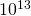that of our Sun and acts like a single mass 0.613 Mpc away? (b) What is the ratio of this force to the person’s weight? Note that Andromeda is the closest large galaxy.

(a) A particle and its antiparticle are at rest relative to an observer and annihilate (completely destroying both masses), creating tworays of equal energy. What is the characteristic-ray energy you would look for if searching for evidence of proton-antiproton annihilation? (The fact that such radiation is rarely observed is evidence that there is very little antimatter in the universe.) (b) How does this compare with the 0.511-MeV energy associated with electron-positron annihilation?

a. 938.27 MeV; b.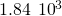The peak intensity of the CMBR occurs at a wavelength of 1.1 mm. (a) What is the energy in eV of a 1.1-mm photon? (b) There are approximately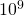photons for each massive particle in deep space. Calculate the energy ofsuch photons. (c) If the average massive particle in space has a mass half that of a proton, what energy would be created by converting its mass to energy? (d) Does this imply that space is “matter dominated”? Explain briefly.

(a) Use the Heisenberg uncertainty principle to calculate the uncertainty in energy for a corresponding time interval of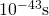. (b) Compare this energy with the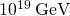unification-of-forces energy and discuss why they are similar.

a.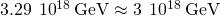; b. 0.3; Unification of the three forces breaks down shortly after the separation of gravity from the unification force (near the Planck time interval). The uncertainty in time then becomes greater. Hence the energy available becomes less than the needed unification energy.

### Glossary

thermal radiation produced by the Big Bang event
dark energy
form of energy believed to be responsible for the observed acceleration of the universe
dark matter
matter in the universe that does not interact with other particles but that can be inferred by deflection of distance star light
nucleosynthesis
creation of heavy elements, occurring during the Big Bang